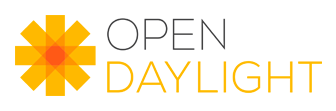author Lukas Sedlak Thu, 28 Mar 2013 12:09:04 +0000 (13:09 +0100) committer Tony Tkacik Thu, 28 Mar 2013 12:15:50 +0000 (13:15 +0100)
Signed-off-by: Tony Tkacik <ttkacik@cisco.com>

index e99bbb1..9103173 100644 (file)
@@ -20,16 +20,23 @@ import org.opendaylight.controller.yang.model.api.TypeDefinition;
* 4</a>). <br>\r
* The canonical form of a binary value follows the rules in <a\r
* href="https://tools.ietf.org/html/rfc4648">[RFC4648]</a>.\r
- *\r
- *\r
+ * \r
+ * <br>\r
+ * <br>\r
+ * This interface was modeled according to definition in <a\r
+ * href="https://tools.ietf.org/html/rfc6020#section-9.8">[RFC-6020] The binary\r
+ * Built-In Type</a>\r
+ * \r
*/\r
public interface BinaryTypeDefinition extends\r
TypeDefinition<BinaryTypeDefinition> {\r
\r
/**\r
* Returns List of number of octets that binary value contains.\r
-     *\r
+     * \r
* @return List of number of octets that binary value contains.\r
+     * \r
+     * @see LengthConstraint\r
*/\r
public List<LengthConstraint> getLengthConstraints();\r
}\r
index 92f3098..5b40c49 100644 (file)
@@ -11,8 +11,30 @@ import java.util.List;
\r
import org.opendaylight.controller.yang.model.api.TypeDefinition;\r
\r
+/**\r
+ * IntegerTypeDefinition is interface which represents SIGNED Integer values\r
+ * defined in Yang language. <br>\r
+ * The integer built-in types in Yang are int8, int16, int32, int64. They\r
+ * represent signed integers of different sizes: <br>\r
+ * <ul>\r
+ * <li>int8 represents integer values between -128 and 127, inclusively.</li>\r
+ * <li>int16 represents integer values between -32768 and 32767, inclusively.</li>\r
+ * <li>int32 represents integer values between -2147483648 and 2147483647,\r
+ * inclusively.</li>\r
+ * <li>int64 represents integer values between -9223372036854775808 and\r
+ * 9223372036854775807, inclusively.</li>\r
+ * </ul>\r
+ * \r
+ * The Integer Built-In Types are defined in <a\r
+ * href="https://tools.ietf.org/html/rfc6020#section-9.2"> [RFC-6020]</a>\r
+ */\r
public interface IntegerTypeDefinition extends\r
TypeDefinition<IntegerTypeDefinition> {\r
\r
+    /**\r
+     * Returns Range Constraints defined for given Integer Type.\r
+     * \r
+     * @return Range Constraints defined for given Integer Type.\r
+     */\r
List<RangeConstraint> getRangeStatements();\r
}\r
index 8c9cb79..97f4448 100644 (file)
@@ -9,9 +9,42 @@ package org.opendaylight.controller.model.api.type;
\r
\r
+/**\r
+ * The Lenght Constraint value consists of an explicit value, or a lower bound\r
+ * returned by {@link #getMin()} and an upper bound returned by\r
+ * <br>\r
+ * Length-restricting values MUST NOT be negative. A length value is a\r
+ * non-negative integer, or one of the special values <code>min</code> or\r
+ * <code>max</code>. The defined <code>min</code> and <code>max</code> mean the\r
+ * minimum and maximum length accepted for the type being restricted,\r
+ * respectively. <br>\r
+ * An implementation is not required to support a length value larger than\r
+ * <br>\r
+ * <br>\r
+ * This interface was modeled according to definition in <a\r
+ * href="https://tools.ietf.org/html/rfc6020#section-9.4.4">[RFC-6020] The\r
+ * length Statement</a>.\r
+ * \r
+ */\r
public interface LengthConstraint extends ConstraintMetaDefinition {\r
\r
+    /**\r
+     * Returns the length-restricting lower bound value. <br>\r
+     * The value MUST NOT be negative.\r
+     * \r
+     * @return the length-restricting lower bound value.\r
+     */\r
Long getMin();\r
\r
+    /**\r
+     * Returns the length-restricting upper bound value. <br>\r
+     * The value MUST NOT be negative.\r
+     * \r
+     * @return length-restricting upper bound value.\r
+     */\r
Long getMax();\r
}\r
index 76bfb75..12fbb1b 100644 (file)
@@ -9,9 +9,37 @@ package org.opendaylight.controller.model.api.type;
\r
\r
+/**\r
+ * The Range Constraint interface is used to restrict integer and decimal\r
+ * built-in types, or types derived from those.\r
+ * <br>\r
+ * A range consists of an explicit value consists of an explicit value, or a lower bound\r
+ * returned by {@link #getMin()} and an upper bound returned by\r
+ * <br>\r
+ * Each explicit value and range boundary value given in\r
+ * the range expression MUST match the type being restricted, or be one of the\r
+ * special values "min" or "max". "min" and "max" mean the minimum and maximum\r
+ * value accepted for the type being restricted, respectively\r
+ * <br>\r
+ * <br>\r
+ * This interface was modeled according to definition in <a\r
+ * href="https://tools.ietf.org/html/rfc6020#section-9.2.4">[RFC-6020] The\r
+ * range Statement</a>.\r
+ */\r
public interface RangeConstraint extends ConstraintMetaDefinition {\r
\r
-    Long getMin();\r
+    /**\r
+     * Returns the length-restricting lower bound value.\r
+     * \r
+     * @return the length-restricting lower bound value.\r
+     */\r
+    Number getMin();\r
\r
-    Long getMax();\r
+    /**\r
+     * Returns the length-restricting upper bound value.\r
+     * \r
+     * @return the length-restricting upper bound value.\r
+     */\r
+    Number getMax();\r
}\r
index acc8a34..0f09842 100644 (file)
@@ -7,6 +7,7 @@
*/\r
package org.opendaylight.controller.yang.model.api;\r
\r
+\r
public interface TypeDefinition<T extends TypeDefinition<T>> extends SchemaNode {\r
\r
T getBaseType();\r